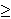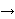## The Strong Circular 5-Flow Conjecture

Let r2 be a real number and let G be a graph and D an orientation of its edges. A function f: E(G)R is a nowhere-zero circular r-flow if for every vertex v of G, the sum of the f-values on the D-incoming edges at v equals the sum of the f-values on the D-outgoing edges at v, and for every edge e of G, the value |f(e)| is between 1 and r - 1. Let us observe that if G admits a nowhere-zero circular r-flow, then it admits an integer valued nowhere-zero k-flow, where k is the `ceiling' of r

The circular flow index of G is the minimum value r for which G admits a nowhere-zero circular r-flow.

The Tutte 5-Flow Conjecture asserts that every 2-edge-connected graph admits a nowhere-zero (circular) 5-flow. Among cubic graphs, snarks are nontrivial graphs whose circular flow number is bigger than four, i.e. they do not admit nowhere-zero 4-flows.

Conjecture 1: Let G be a snark distinct from the Petersen graph. Then the circular flow index of G is less than 5.

Conjecture 1 has been verified for some small snarks.

It is not difficult to see that the circular flow index of the Petersen graph is equal to 5. So, Conjecture 1 claims that this is the only snark with that high circular flow index.

Maybe Conjecture 1 can be strengthened as follows (although I do not believe this is true, so I sustain of conjecturing it).

Problem 2: Is it true that there exists a constant r < 5 such that every cyclically 4-edge-connected graph distinct from the Petersen graph admits a nowhere-zero circular r-flow?